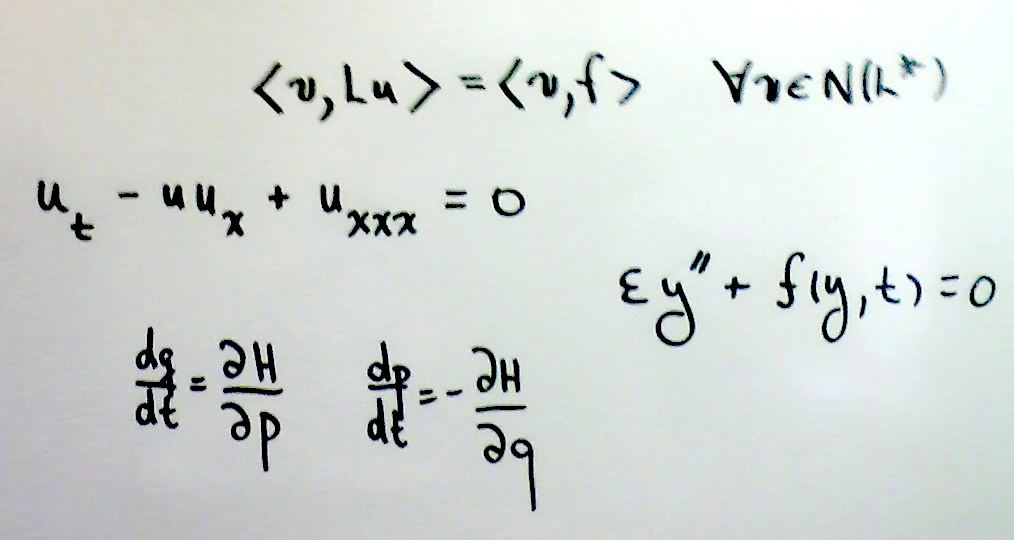## Math 560/561  -  Applied Math I/II *Textbook Principles of Applied Mathematics (1rst or 2nd ed.), James Keener (not required but highly recommended)
Instructor Mark Pernarowski
Grading Your grade will be based strictly on homework assignments.  The raw score for each assignment will vary. If P is the percentage of the sum of all the raw scores then your letter grade will be determined from:

 A A- B+ B B- C+ C C- D F 90-100 87-89 84-86 80-83 77-79 74-76 70-73 67-69 60-66 0-59

Assignments must be written in a clear and logical fashion.Homework Homework 1 :Inner product spaces, diagonalization, Fredholm applications, Least Sq. Due: Thursday, Sept 22 Homework 2: Operator boundedness, eigenfunctions, adjoint calculations Due: Thursday, Oct 13 Homework 3: Operators, Fredholm alternative and Distributions Due: Thursday, Nov 3-7 Homework 4: Green's functions and Sturm Liouville Due: Nov 17-22 Homework5:  Euler Lagrange Eqns, Natural BC, Extrema, Geodesics Due: Thursday, Feb 2 Homework 6: Isoperimetric problems, Transversaiity, Convexity, Several independent                     variables, Lagrangian Mechanics. Due: Thursday, Feb 23 Homework 7: Regular perturbation approximations and intro to asymptotics Due: Thursday, Mar 30 Homework_8: Singular Boundary Value Problems Due: Thursday, Apr 20Notes Inner product spaces, bases, coordinates, Gram Schmidt, Least Squares Eigenvalues, self adjoint, diagonalization, maximum principle Subspaces, Projections and the Fredholm Alternative for Matrices Least Squares and Normal Equations Hilbert Spaces and Operators Functional 101, Adjoints and Fredholm Alternative Operator Inverses Distributions                         (useful old notes here) Greens functions Sturm Liouville Theory Sturm Liouville Applications Calculus of Variations - Introduction Calculus of Variations - Theory, issues, NBC, L(x,y,y') and L(x,y,y',y'') Calculus of Variations - Multi-variable L(x,y1,y1',y2,y2',...) introduction Calculus of Variations - Geodesics and Isoperimetric Problems and Transversality Calculus of Variations - Convexity, Sufficiency, Existence and Convexity overview Calculus of Variations - Several independent variables. Calculus of Variations - Least Actions and Hamiltonian Mechanics Perturbation Theory - Intro Perturbation Theory - Regular         (Implicit Function Theorem) Perturbation Theory - Asymptotics Perturbation Theory - Asymptotic root approximations Perturbation Theory - Matching Theory and Concepts Perturbation Methods - BVP Perturbation Methods - Oscillations and Multiple Scales  MS-systems Perturbation Methods - Adiabatic Invariants Perturbation Methods - Averaging (big) Perturbation Methods - Kuzmak-Luke Strongly nonlinear osc.Supplemental Operator Theory Summary Sheet  and Compact Operators Boundary Value Problems - 2nd order Integral Equation examples Functional Analysis - Bounded Operators Functional Analysis - Fredholm Alternative Functional Analysis - Mixed with Integral equations Learning Outcomes: Upon completion of the course students will have an understanding of the following:Inner product spaces: Bases, coordinates, Gram Schmidt orthogonalization, Least squares approximations Matrices: adjoints, diagonalization, maximum principle, projections, Least squares solutions, singular value decomposition, normal equations and the Fredholm alternative Introductory theory of Hilbert spaces including an overview of the properties of bounded, unbounded, and compact operators and their adjoints. Completeness and spectral properties of self adjoint operators. Fredholm alternative and applications. Operator inverses. Introduction to distributions on test function spaces and their properties. Green's functions and Sturm Liouville theory for Boundary Value Problems. Calculus of Variations introduction: functionals, admissible sets, natural boundary conditions for Lagrangians of single and several dependent variables. Euler Lagrange equation derivations Intermediate Calculus of Variation topics: geodesics, isoperimetric problems, transversality, Lagrangians with several independent variables, convexity and sufficiency conditions for minima. Lagrangian and Hamiltonian mechanics and the principle of Least action. Regular perturbation theory for algebraic and differential systems. Implicit function Theorem. Asymptotic expansions and series with applications to root approximation. Matching Theory for asymptotic expansions, overlap domains, intermediate variables. Matched asymptotic expansions for boundary value problems. Perturbation techniques for oscillatory systems: Multiple scales approaches with different temporal straining, the method of averaging and adiabatic invariants.